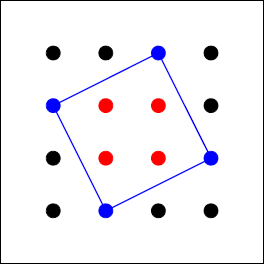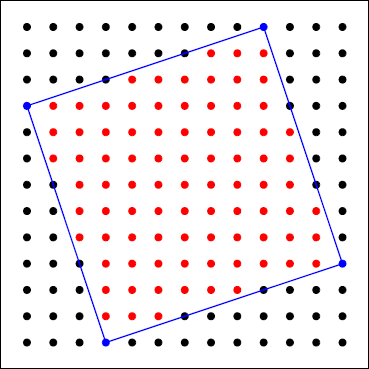### Problem Statement

Find the area of the minimum square in the plane, such that its 4 vertices are distinct lattice points and there are exactly n lattice points strictly inside it. If there is no such square, return 0 instead.

### Definition

 Class: EnclosingSquare Method: getMinimum Parameters: long Returns: long Method signature: long getMinimum(long n) (be sure your method is public)

### Notes

-A lattice point in the coordinate plane is a point whose x-coordinate and y-coordinate are both integers.

### Constraints

-n will be between 0 and 1,000,000,000,000, inclusive.

### Examples

0)

 `4`
`Returns: 5`
 The square below contains exactly 4 lattice points strictly inside itself and has an area of 5.1)

 `3`
`Returns: 0`
 There is no such square.
2)

 `1000000000000`
`Returns: 1000000000001`
3)

 `101`
`Returns: 104`
4)

 `85`
`Returns: 90`#### Problem url:

http://www.topcoder.com/stat?c=problem_statement&pm=11044

#### Problem stats url:

http://www.topcoder.com/tc?module=ProblemDetail&rd=14287&pm=11044

lyrically

#### Testers:

SnapDragon , Rustyoldman , marek.cygan , timmac , ivan_metelsky , Egor

Math